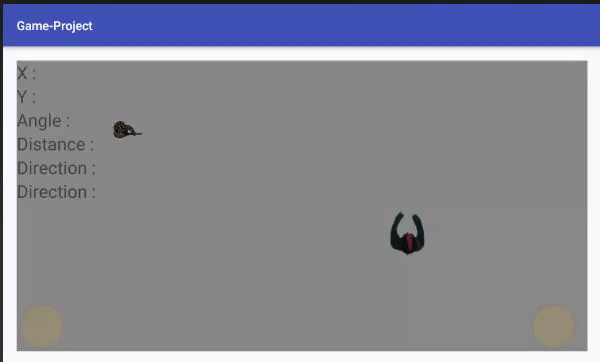# My Zombie will not be found.

That sounds extremely philosophical doesn't it?

Anyways, I have a rather complex problem.

My main_activity class gathers all of the Zombies like so:

``````//Testing Runnable (used to compare the first zombie with the player)
private Runnable updateLocations = new Runnable(){
@Override
public void run(){
try {
while(true) {
image_player.getLocationInWindow(pLoc);
Zombie zoms = zombieCollection.next();
if(!zoms.equals(null)){
zoms.getZombieImage().getLocationInWindow(zLoc);
}
System.out.println("Zombie: x = " + zLoc + "; y = " + zLoc);
System.out.println("Player: x = " + pLoc + "; y = " + pLoc);
}
} catch (InterruptedException e) {
e.printStackTrace();
}
}
};
```
```

My zombie class gathers information like so:

``````public class Zombie{

float X, Y;
int Width, Height;
Direction fdirc;
ImageView zImage;
Player player;

int[] zLoc;

public Zombie(ImageView zImage, Player player){
zLoc = new int;
zImage.getLocationOnScreen(zLoc);

this.zImage = zImage;
this.X = zLoc;
this.Y = zLoc;
this.Width = zImage.getWidth();
this.Height = zImage.getHeight();
this.fdirc = Direction.EAST;
this.player = player;

}

public ImageView getZombieImage(){
return zImage;
}
private Runnable startZombieChase = new Runnable() {
@Override
public void run() {
try {
moveTowardsPlayer();

updateZombie.sendEmptyMessage(0);
}
} catch (InterruptedException e) {
e.printStackTrace();
}
}
};
private Handler updateZombie = new Handler(Looper.getMainLooper()) {
public void handleMessage(android.os.Message msg) {

/** Because the zombie should always be on top! **/
zImage.getLocationOnScreen(zLoc);
zImage.bringToFront();
zImage.setX(X);
zImage.setY(Y);

}
};

private void moveTowardsPlayer(){
int player_x = player.getPosition();
int player_y = player.getPosition();

l("Where is it in zombie class : player - " + player_x + " " + player_y + "zombie  - " + X + " " + Y);

float compareX = player_x - (int)X;
float compareY = player_y - (int)Y;

// Y is closer, so we're moving horizontally.
if(Math.abs(compareX) < Math.abs(compareY)){
//Moving North
if(player_y > Y){
Y+=1;
}
//Moving South
else if(player_y < Y){
Y-=1;
}
}
// X is closer, so we're moving vertically.
else{
//Moving East
if(player_x > X){
X+=1;
}
//Moving West
else if(player_x < X){
X-=1;
}

}
}
public void l(Object string){
System.out.println("Log - " + string);
}
}
```
```

The problem I'm having is that it will move relative to a number (So, it does move relative to something) but, not the correct thing.logcat tells me this:

• Where is it in zombie class: player - 750 451 zombie - 750 451
• Where it is in main_activity: player - 750 451 zombie - 792 619

Could anyone help me understand what I'm doing wrong? The entire project is located here.

Zombies that moves away from the Brainz, must be an ill Zombie. We can't have that, now can we?

To have a function that moves the Zombie towards the non-zombie, you use a function, but that function makes use of variables that are not arguments, and there fore hard to find out where they come from. I'd go with something like this: (this is a bit verbose, but clearly shows what is happening)

``````/*
* Function to update the position of the Zombie, aka walk to the Player.
* @player_pos       - Where's the Brainz at?
* @zombie_pos       - Where am I?
* Might want to build it overloaded with an option for the speed.
*
* @return           - We return the new Zombie pos.
*/
private double [] moveTowardsPlayer(double [] player_pos, double [] zombie_pos) {
// To make sure we don't override the old position, we copy values. (Java stuff)
double [] player_pos_old = player_pos.clone();
double [] zombie_pos_old = zombie_pos.clone();

// Let's get the new X pos for the Zombie
double left_or_right = player_pos_old - zombie_pos_old; // pos number is right, neg is left
double zombie_pos_new_x;
if (left_or_right > 0) { // Right
zombie_pos_new_x = player_pos_old + zombie_speed;
} else { // Left - this way we make sure we are always getting nearer to the Brainz.
zombie_pos_new_x = player_pos_old - zombie_speed;
}

// TODO: do the same for the Y pos.

// Bring it together
double [] zombie_pos_new = {zombie_pos_new_x, zombie_pos_new_y};

// One step closer to the Brainz!
return zombie_pos_new;
}
```
```

And use like:

``````double [] zombie_pos = moveTowardsPlayer([2, 2], [5, 4]);
this.X = zombie_pos; // I'd advice to keep these together as one var.
this.Y = zombie_pos; // But it's your game.
```
```

And then figure out when the Zombie gets the Brainz (or the Bullet)